### Home > CALC > Chapter 5 > Lesson 5.5.2 > Problem5-173

5-173.
1. Use the definition of the derivative to write an expression (i.e. the limit of a difference quotient) representing each of the following derivatives. Then evaluate each limit to find the actual derivative. L'Hôpital's Rule may be used. Homework Help ✎

1. f ′(x), where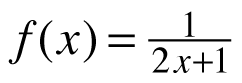.

2. h ′(x), where h(x) = (x − 2)−2.

3. g ′(4), where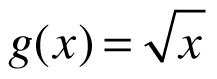.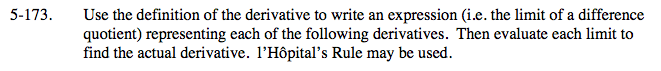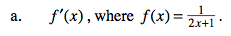Setup the definition of the derivative as a limit.
This is Hana's Method:

Make a choice about how to evaluate this limit. These are your choices:
1) Use Algebra. Simplify the fraction until you can 'cancel out' the h in the denominator and then evaluate as h→0.
2) Deconstruct Hana's Method. Once you identify f(x), find f '(x) using the Power Rule. Of course, in this case, that is working in circles.
3) Use l'Hôpital's Rule; after all, there is a 0 in the numerator and the denominator.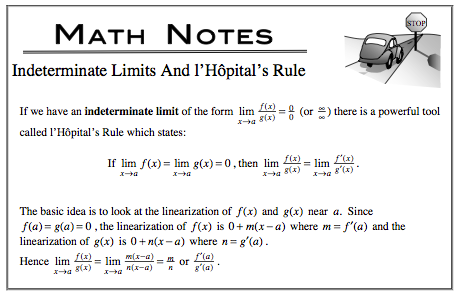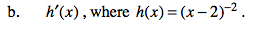Refer to the hints in part (a).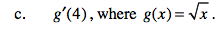Refer to the hints in part (a).